# PHASE DIAGRAMS Phase Diagrams u Temperature and pressure

• Slides: 15PHASE DIAGRAMSPhase Diagrams u Temperature and pressure control the phase of a substance. u A phase diagram is a graph of pressure versus temperature that shows in which phase a substance exists under different conditions of temperature and pressure.Phase Diagrams u. A phase diagram typically has three regions, each representing a different phase and three curves that separate each phase.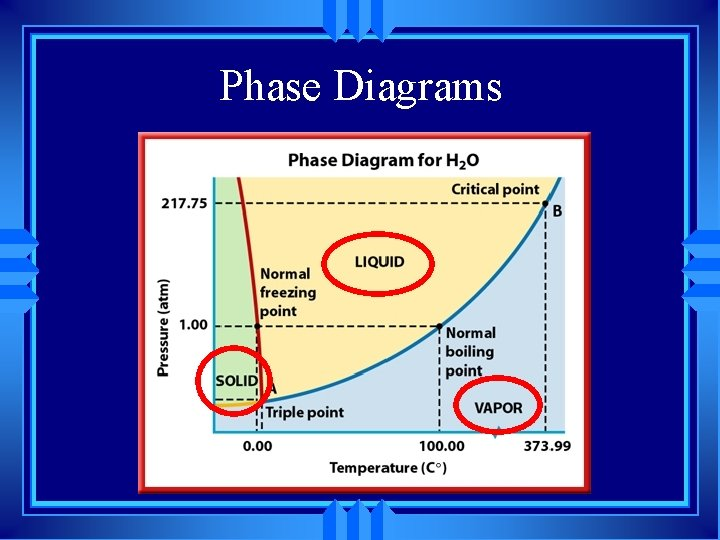Phase Diagrams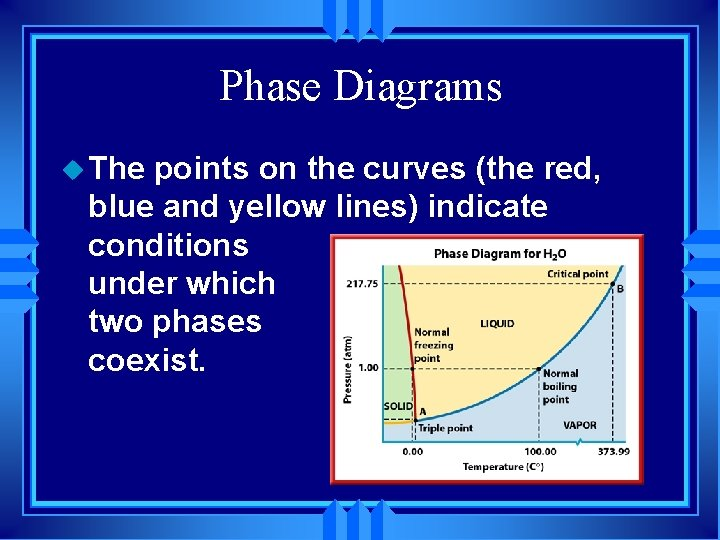Phase Diagrams u The points on the curves (the red, blue and yellow lines) indicate conditions under which two phases coexist.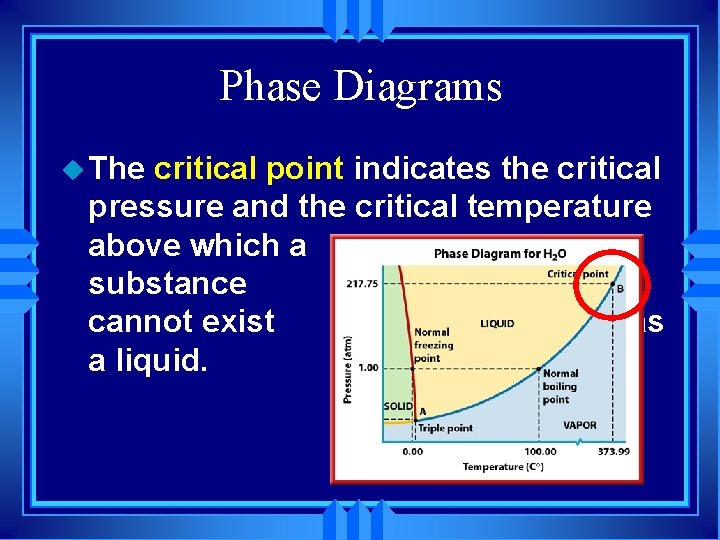Phase Diagrams u The critical point indicates the critical pressure and the critical temperature above which a substance cannot exist as a liquid.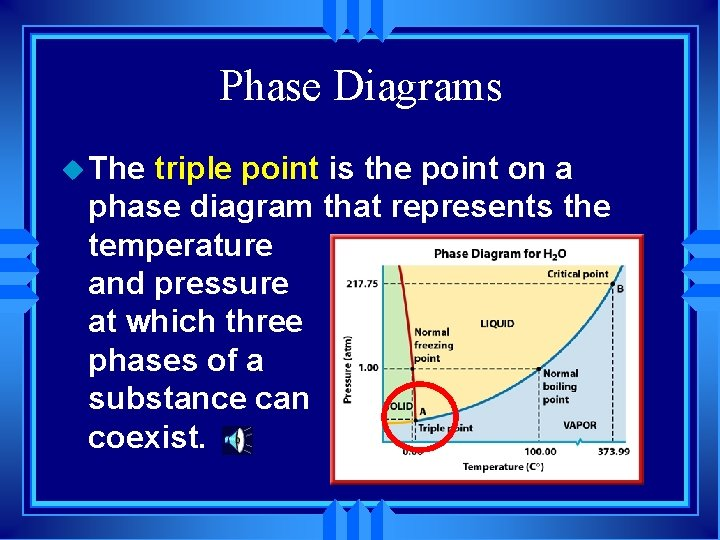Phase Diagrams u The triple point is the point on a phase diagram that represents the temperature and pressure at which three phases of a substance can coexist.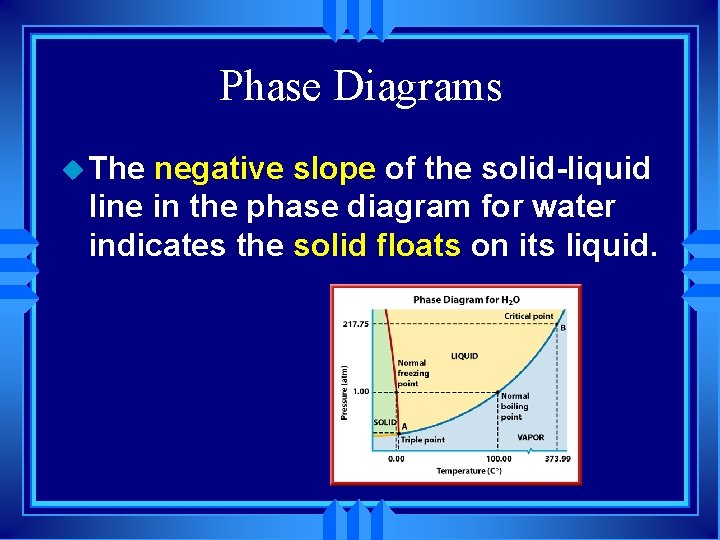Phase Diagrams u The negative slope of the solid-liquid line in the phase diagram for water indicates the solid floats on its liquid.Question 1 What phase change occurs for carbon dioxide at -100 ºC and 1 atm pressure as it is heated to room temperature? (The solid CO 2 sublimes to CO 2 gas. )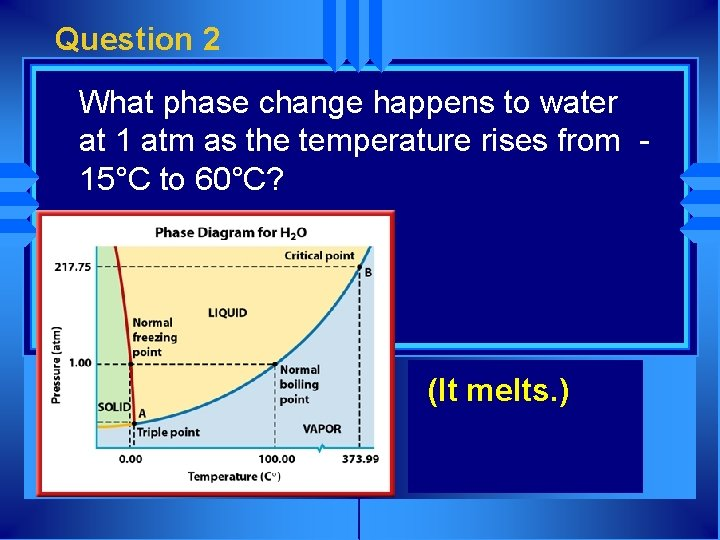Question 2 What phase change happens to water at 1 atm as the temperature rises from 15°C to 60°C? (It melts. )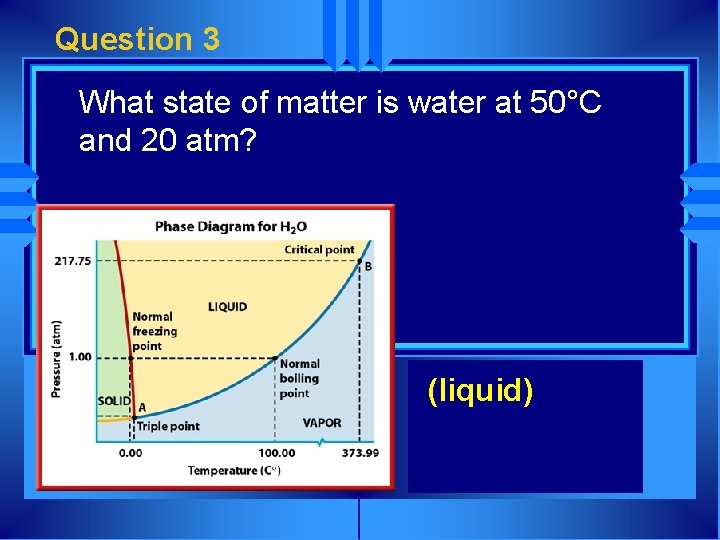Question 3 What state of matter is water at 50°C and 20 atm? (liquid)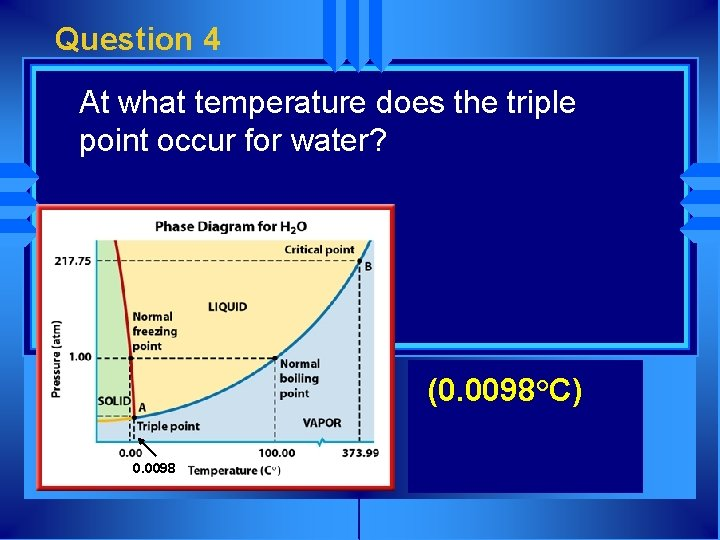Question 4 At what temperature does the triple point occur for water? (0. 0098°C) 0. 0098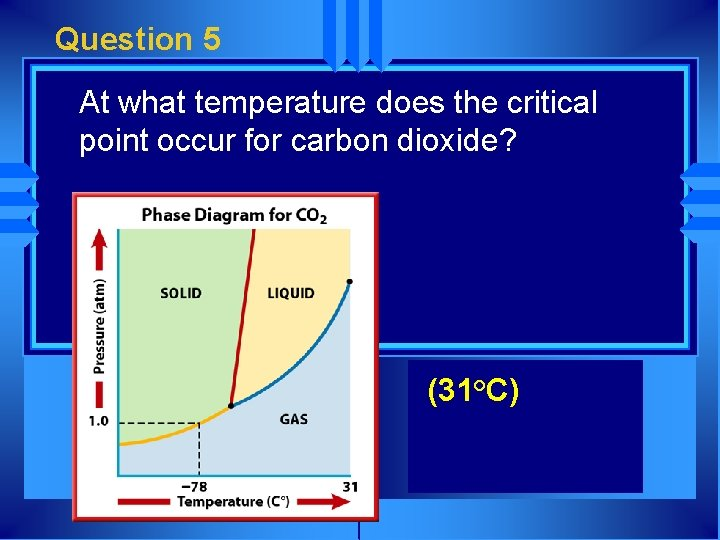Question 5 At what temperature does the critical point occur for carbon dioxide? (31°C)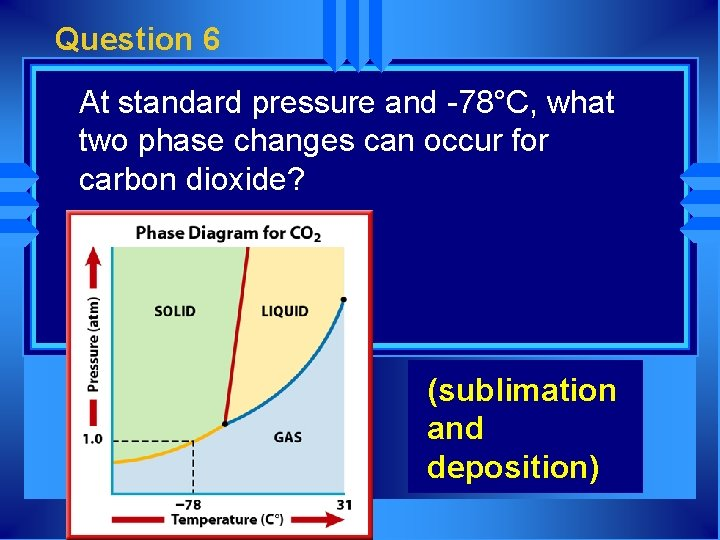Question 6 At standard pressure and -78°C, what two phase changes can occur for carbon dioxide? (sublimation and deposition)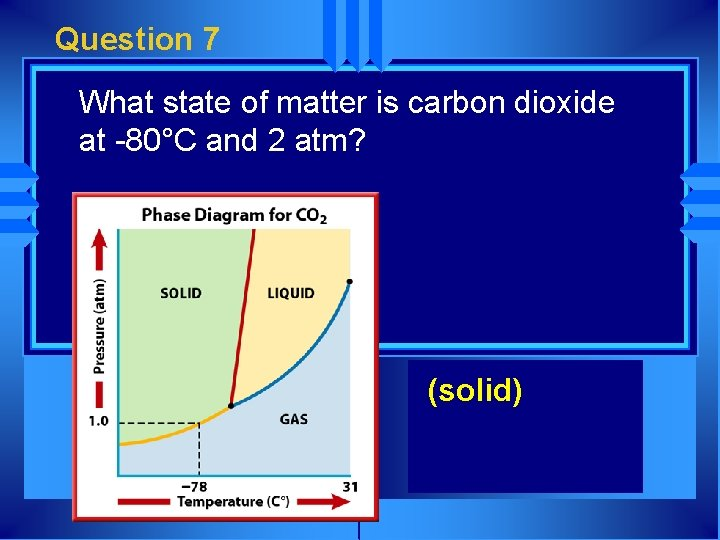Question 7 What state of matter is carbon dioxide at -80°C and 2 atm? (solid)